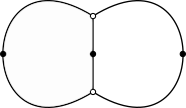## 7CCMMS16T -- Algebraic Curves• Office: S5.29
• Office hours: Fri 14:00-16:00
• Lecture times and place (begins on 27 Sep.): Fri 9:00-11:00 STRAND S5.20
• Tutorials (begins on 4 Oct.): Fri 11:00-12:00 STRAND S5.20
• References: These are some of the books that I will be drawing from for the lectures
• Simon Donaldson, Riemann Surfaces
• Ernesto Girondo, Gabino González-Diez, Introduction to Compact Riemann Surfaces and Dessins d'Enfants
• Frances Kirwan, Complex algebraic curves
• Send me an e-mail if you are going to continue with this course.

### Lecture notes

Here are the lecture notes. They are mostly taken from the above references. I will be updating these as we go along.

### Homework

Homework will appear here.

### Past Exams

Please don't worry too much about the exam. Focus on learning the material and you will be fine. I have included hand-written solutions to Exam 2019 to give you an idea of how to organize your solutions.

### Computer Experiments

Here I plan to record various code snippets. I encourage you to experiment with these in order to get a feel for the material of the course.
This is strictly experimental and non-examinable material. I plan to use SageMath (a free open-source mathematics software licensed under GPL).
You can either install it on your computer or if you just want to check out how it works, you can use the sagecell given in the bottom of this page.
Feel free to submit code that would go here by e-mail. I'll post it here with an acknowledgment.

• Draw algebraic curves over real numbers (RR). Here is an elliptic curve:

 R.<x,y>=AffineSpace(RR, 2) C = Curve([y^2 - x^3 +x], R) C.plot() 
Try different curves. Here are some interesting ones: y^2+x^4-x^2 (eight curve), y^4+x^4-1 (square),  y^2-x^3-7 (the Bitcoin curve), (x^2+y^2-1)^3+27*x^2*y^2 (astroid).

Or you can draw a random one (of degree 4 over rationals QQ) as follows:

 P.<x,y> = PolynomialRing(QQ) p = P.random_element(degree=4, terms=15) print p R.<x,y> = AffineSpace(QQ, 2) C = Curve(p, R) C.plot() 
You maybe lucky and get a curve like this one that I got

 x^4 - x^2*y^2 + y^4 - x^3 - 5*x^2 + 17/539*y^2 + x - 7/3

For some unexplainable reason I get happy when I get a compact curve.

• Check if a polynomial of two variables (over complex numbers CC) is reduced (square-free).

 P.<x,y>=PolynomialRing(CC) f = x^4+x*y^3+x^3*y+y^4 gcd([f,f.derivative(x),f.derivative(y)]) 
If gcd is not a constant, then the polynomial is not reduced. To find the reduced polynomial replace the last line with

 f//gcd([f,f.derivative(x),f.derivative(y)]) 
(Working over CC, Sage does not always simplify the constants appearing in the computation. This is not important as we only care about polynomials up to constant factors.)

• Check if an algebraic curve defined by a polynomial is singular (over complex numbers).

 R.<x,y>=AffineSpace(CC, 2) C = Curve([(x^2+y^2-1)^3+27*x^2*y^2], R) C.is_singular() 

and find the singular points

 C.singular_points() 

• This computes the Taylor expansion at a point (x_0,y_0) = (3,-1) up to degree d =10

 x,y = var("x y") taylor(x^2*y + x*y^2 - 4*x*y + x + 9*y, (x,3), (y,-1),10) 

• This empty spot is waiting for you to submit code that computes the multiplicity and the tangent cone of a singularity.  
• This empty spot is waiting for you to submit code that expresses a homogeneous polynomial in two variables as a product of linear forms.  
• Here is how you draw the Newton polytope of a polynomial

 R.<x,y>=AffineSpace(CC, 2) p = CC(3+4*I)*x^2 + CC(1+I)*x*y + y + x - x^2*y + 3*y^3 P= p.newton_polytope() ; P 

and here is how you can see the Minkowski sum of two polyhedra

 X = Polyhedron(vertices=[(0,1),(1,0),(0,0),(1,1)]) Y = Polyhedron(vertices=[(0,0), (0,1), (2,3)]) X+Y 

• The following code determines whether a bivariate square-free polynomial p is irreducible over the complex numbers. The number of irreducible components of p is given by the output of .
This code uses quite sophisticated techniques that go beyond the scope of this course but it could be useful as a blackbox which is why it is included here.

 singular.lib('deRham.lib') R = singular.ring('0','(x,y)','dp') p = singular('x^6+x^2*y^4+y^2*x^4+y^6') L = singular.list([p]) singular.deRhamCohomology(L); 

• Compute the projectivization of an affine curve

 R.<x,y>=AffineSpace(CC, 2) C = Curve(y - x^3 + x - 1, R) C.projective_closure() 

• Compute the affine patch of a projective curve

 P.<x,y,z> = ProjectiveSpace(CC, 2) C = Curve(x^3 - x^2*y + y^3 - x^2*z, P) C.affine_patch(0) 
Use  C.affine_patch(1)  or  C.affine_patch(2) for other patches.